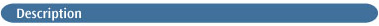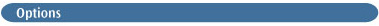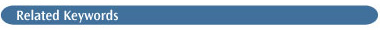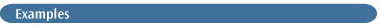# GuessThis keyword controls the initial guess for the Hartree-Fock wavefunction. Guess is not meaningful without an option. By default, a Harris guess is used (see below).Harris
Diagonalize the Harris functional  for the initial guess. This is the default unless atoms heavier than Xe are present.

Huckel
Requests that a Huckel guess be generated, which is the default when atoms heavier than Xe are present.

RdScale
Read in the scale factor on atomic hardnesses used in iterative extended Huckel. The default is 7.0 times the QEq value.

OldHuckel
Use the old Huckel guess (pre-Gaussian 03) instead of CNDO or the updated Huckel.

INDO
Use the Gaussian 98 default guess: INDO for first-row systems, CNDO for second-row,and Huckel for third-row and beyond.

AM1
Do an AM1 calculation for the initial guess (currently only works with sparse matrix code). Guess=(AM1,Always) causes later steps in a geometry optimization to generate a new guess at each point and compare the energies with the density from the old point and the new guess and take the better.

Core
Requests that the core Hamiltonian be diagonalized to form the initial guess. Guess=Core is most commonly used for atomic calculations.

DensityMix[=N]
Whether to mix occupied and virtual orbital contributions in forming the initial guess density. N defaults to -3 (use Huckel eigenvalues to decide which orbitals to mix).

Permute
Read in a permutation of orbitals in the initial guess. The numbers of the generated guess orbitals are given in the order in which they should be used in the SCF. Ranges (e.g. 7-12) can be used, and all orbitals not listed are put in after the listed orbitals in their original order. Separate permutation lists for α and β orbitals must be specified (each list separated by a blank line) for open shell systems.

Alter
Indicates that the orbitals selected for occupation in the Hartree-Fock wavefunction should not be those of lowest energy. Normally, the occupied orbitals are selected as those with lowest eigenvalues for the one-electron Hamiltonian used in the initial guess programs. The alteration sections consist of a set of transpositions indicating that one of these occupied orbitals is to be replaced by one of the other (virtual) orbitals. Each such transposition is on a separate line and has two integers N1 and N2 (free format, separated by spaces or a comma as usual) indicating that orbital N1 is to be swapped with orbital N2. The list of orbital transpositions is terminated by the blank line at the end of the input section.

For UHF calculations, two such orbital alteration sections are required, the first specifying transpositions ofα orbitals, and the second specifying transpositions of β orbitals. Both sections are always required. Thus, even if only α transpositions are needed, the β section is required even though it is empty (and vice-versa). The second blank line to indicate an empty β section must be included.

Read
Requests that the initial guess be read from the checkpoint file (Guess=Read is often specified along with Geom=Checkpoint). This option may be combined with Alter, in which case the orbitals are read from the checkpoint file, projected onto the current basis set, and then the specified alterations are made. Checkpoint is a synonym for Read. The TCheck option says to attempt to read a guess from the checkpoint file, but to generate a new one if necessary.

Always
Requests that a new initial guess be generated at each point of an optimization. By default, the SCF results from the last point are used for the guess at the next point.

Mix
Requests that the HOMO and LUMO be mixed so as to destroy α-β and spatial symmetries. This is useful in producing UHF wavefunctions for singlet states.

LowSymm
Requests that irreducible representations of the molecular point group be combined in the symmetry information used in the N3 steps in the SCF, to allow lowered symmetry of the wavefunction. This enables the orbitals (and possibly but not necessarily the total wavefunction) to have lower symmetry than the full molecular point group. This option is available only for GVB calculations, where it is often necessary for calculations on symmetric systems (see the discussion of the GVB keyword below for an example using this option).

The option expects a single line of input (in the format 16I2) giving the numbers of the irreducible representations to combine, with the new groups separated by 0; the list itself must be terminated by a 9. The numbers correspond to the order in which the representations are listed by Link 301 in the output file (see the examples subsection below).

Since this input section is always exactly one line long, it is not terminated by a blank line. Note that irreducible representations are combined before orbital localization is done and that localized orbitals retain whatever symmetry is kept. Guess=NoSymm removes all orbital symmetry constraints without reading any input.

NoSymm
Requests that all orbital symmetry constraints be lifted. Synonymous with SCF=NoSymm and Symm=NoSCF.

Local
Requests that orbitals be localized using the Boys method . Occupied and virtual orbitals are localized separately, and the irreducible representations (after possible merging using LowSymm or NoSymm) are not mixed. Localized orbital analysis of a converged SCF wavefunction may then be done using a second job step, which includes Guess(Read,Local,Only) and Pop=Full in its route section.

Translate
Translate requests that the coordinates of the atoms used to produce a guess, which is read in, be translated to the current atomic coordinates. This is the default. It may fail in unusual cases, such as when a wavefunction is used as a guess for a system with a different stoichiometry, in which case Guess=NoTranslate should be specified.

Cards
Specifies that after the initial guess is generated, some or all of the orbitals will be replaced with ones read from the input stream. This option can be used to read a complete initial guess from the input stream by replacing every orbital. The replacement orbitals are placed in the input section following the guess alteration commands, if any. For UHF, there are separate α and β replacement orbital input sections.

The replacement orbitals input section (the α replacement orbitals section for UHF) begins with a line specifying the Fortran format with which to read the replacement orbital input, enclosed in parentheses. For example: (4E20.8). The remainder of the section contains one or more instances of the following:

```IVec                                          Orbital to replace (0=end, -1=replace  all orbitals in order).
(A(I,IVec),I=1,N)                                New orbital in the format specified in the first line.```

The format for the line containing IVec is Fortran I5. The β orbital replacement section for UHF calculations differs only in that it omits the initial format specification line. See the examples section for sample replacement orbital input.

Only
Guess=Only functions as a calculation type keyword and requests that the calculation terminate once the initial guess is computed and printed. Note that the amount of orbital information that is printed is controlled by the Pop keyword. Guess=Only may not be used with semi-empirical methods.

This option is useful in preliminary runs to check if configuration alteration is necessary. For example, Guess=Only may be specified with CASSCF in order to obtain information on the number of CI configurations in the CAS active space (as well as the initial orbitals).

Guess(Only,Read) may also be used to produce population and other post-calculation analyses from the data in a checkpoint file. For example, these options alone will produce a population analysis using the wavefunction in the checkpoint file. Guess(Only,Read) Prop will cause electrostatic properties to be calculated using the wavefunction in the checkpoint file.

Save
Save the generated initial guess back into the checkpoint file at the conclusion of a Guess=Only run. This option is useful for saving localized orbitals.

Print
Print the initial guess.

Alpha
Use alpha orbitals for both alpha and beta guess during Guess=Read.

Fock
Reuse Fock matrices rather than orbitals when reading from previous results on the rwf or chk files. This is the default for periodic boundary conditions calculations if Guess=Alter is not specified. NoFock disables this behavior, and it is the default for non-PBC calculations.

Extra
Do an extra, new initial guess when reading orbitals from the RWF (i.e., during geometry optimizations). By default, this is done if the default Harris guess is allowed, no alteration of configuration was requested, and the optimization did not take a small step as flagged by variable 4 in ILSW. Use NoExtra to disable this feature.

ForceAbelianSymmetry
Force the initial guess orbitals to transform according to irreps of the Abelian point group. NoForceAbelianSymmetry is the default.

Sparse
Perform a sparse SE calculation for the initial guess. This option may be useful for very, very large HF or DFT calculations using the sparse matrix facility.

NaturalOrbitals
Include natural orbitals in the checkpoint file. This must be accomplished via a separate job step specifying this option as well as Only and Save. See the discussion of the Population keyword for details.

These options may be combined in any reasonable combination. Thus Guess=(Always,Alter) and Guess=(Read,Alter) work as expected (in the former case, alterations are read once and the same interchanges are applied at each geometry). Conversely, Guess=(Always,Read) is contradictory and will lead to unpredictable results. Refer to the input sections order table at the beginning of this chapter to determine the ordering of the input sections for combinations of options like Guess=(Cards,Alter).

### RESTRICTIONS

Guess=Only may not be used with semi-empirical methods.Transposing 2 Orbitals with Guess=Alter. This example finds the UHF/STO-3G structure of the 2A1 excited state of the amino radical. First, a Guess=Only calculation is run to determine whether any alter instructions are needed to obtain the desired electronic state. The HF/STO-3G theoretical model is used by default:

``` # Guess=Only Test

Amino radical test of initial guess

0 2
n
h 1 nh
h 1 nh 2 hnh

nh 1.03
hnh 120.0      ```

Here is the orbital symmetry summary output from the job, which comes immediately before the population analysis in the output:

```Initial guess orbital symmetries.
Alpha Orbitals:
Occupied (A1) (A1) (B2) (B1) (A1)
Virtual  (A1) (B2)
Beta Orbitals:
Occupied (A1) (A1) (B2) (A1)
Virtual  (B1) (A1) (B2)
<S**2> of initial guess= .7544 ```

Since a doublet state is involved, α and β orbitals are given separately. From the orbital symmetries, the electron configuration in the initial guess is 2a12a12b22a11b1, yielding a 2B1 wavefunction. This is indeed the ground state of NH2. The expectation value of S2 for the unrestricted initial guess is printed. In this case, it is close to the pure doublet value of 0.75.

Note that the orbital energies printed in a Guess=Only job are simply -1.0 for the occupied orbitals and 0.0 for the virtual orbitals, since no SCF has been performed. If the actual orbital energies are desired, a full semi-empirical energy calculation can be performed specifying the desired method (e.g. INDO).

Returning to our consideration of the amino radical, since we want to model the 2A1 excited state, we will need to alter this initial orbital configuration: a β electron must be moved from orbital 4 to orbital 5 (the electron configuration is then 2a12a12b22b11a1).

Guess=Alter may also be used to accomplish this. Here is the input for the geometry optimization

```# UHF/6-31G(d) Opt Guess=Alter Pop=Reg Test

Amino radical: HF/6-31G(d) structure of 2-A1 state

0 2
n
h 1 nh
h 1 nh 2 hnh
Variables:
nh 1.03
hnh 120.0
Blank line ends the molecule specification section.
Blank line ends the α section(empty in this case).
4 5                            Transpose orbitals 4 and 5.
End of the β alteration section. ```

Note that an extra blank line—line 12—is necessary to indicate an empty α alteration section. The final two lines then constitute the β alteration section.

The initial guess program prints a list of orbitals that were interchanged as a result of the Alter option:

```
Projected INDO Guess.
NO ALPHA ORBITALS SWITCHED.
PAIRS OF BETA ORBITALS SWITCHED:
4 5 ```

The eigenvalue of S2 is printed for the UHF wavefunction. The value which results if contamination of the wavefunction from the next possible spin multiplicity (quartets for doublets, quintets for triplets, etc.) is removed is also printed:

```Annihilation of the first spin contaminant:
S**2 before annihilation        .7534,     after     .7500 ```

Although this calculation does in fact converge correctly to 2A1 state, it sometimes happens that the order of orbital symmetries switches during the course of the SCF iterations. If the orbital symmetries of the final wavefunction are different from those in the initial guess (whether or not you are using Guess=Alter), we recommend using the direct minimization routine, specified with the SCF=QC or SCF=DM keywords, which usually holds symmetry from one iteration to the next.

Reordering Orbitals with Guess=Permute. This option is often is the easiest way to perform a complex modification of the initial guess, as in this example:

```# CASSCF/6-31G(d,p) Opt Guess=Permute Pop=Reg Test

CAS job

0 1
molecule specification

1-60 65 63 64 66 68 67 61-62 69     Specify new ordering. ```

Here we have rearranged orbitals 61-68. Listing the final orbital (69) is not really necessary, but it help to make the input easier to understand for humans.

Reading in Orbitals with Guess=Cards. Some or all of the orbitals may be replaced after the initial guess is generated using Guess=Cards. Here is some sample input for this option, which replaces orbitals 1 and 4 (note that the format for the third and following lines is specified in line 1):

```(3E20.8)
1
0.5809834509E+00      0.4612416518E+00    -0.6437319952E-04
0.1724432549E-02      0.1282235396E-14     0.5417658499E-13
0.1639966912E-02     -0.9146282229E-15    -0.6407549694E-13
-0.4538843604E-03      0.6038992958E-04    -0.1131035485E-03
0.6038992969E-04     -0.1131035471E-03
4
0.7700779642E-13      0.1240395916E-12    -0.3110890228E-12
-0.4479190461E-12     -0.1478805861E-13     0.5807753928E+00
0.6441113412E-12     -0.3119296374E-14     0.1554735923E+00
-0.1190754528E-11      0.2567325943E+00     0.1459733219E+00
-0.2567325943E+00     -0.1459733219E+00
0 ```

An orbital number of zero ends the replacement orbital input.